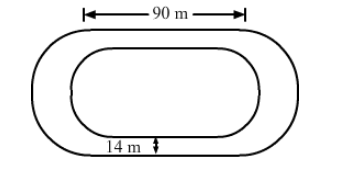# The inside perimeter of a running track shown in the figure is 400 m.`
Question:

The inside perimeter of a running track shown in the figure is 400 m. The length of each of the straight portions is 90 m, and the ends are semicircles. If the track is 14 m wide everywhere, find the area of the track. Also, find the length of the outer boundary of the track.Solution:

Lenath of the inner curved portion $(400-2 \times 90)=220 \mathrm{~m}$

$\therefore$ Length of each inner curved path $=\frac{220}{2}=110 \mathrm{~m}$

​Thus, we have:

$\Rightarrow \pi \mathrm{r}=110$

$\Rightarrow \frac{22}{7} \mathrm{r}=110$

$\Rightarrow r=\frac{110 \times 7}{22}$

$\Rightarrow r=35 \mathrm{~m}$

Outer radius = (35 + 14) = 49 m

Area of track $=\{$ Area of the two rectangles [each $(90 \times 14) \mathrm{m}]+$ Area of the circular ring with $\mathrm{R}=49 \mathrm{~m}$ and $\mathrm{r}=35 \mathrm{~m})\}$

$=(2 \times 90 \times 14)+\frac{22}{7} \times\left[(49)^{2}-(35)^{2}\right]$

$=2520+\frac{22}{7} \times(2401-1225)$

$=2520+\frac{22}{7} \times 1176$

$=2520+3696$

$=6216 \mathrm{~m}^{2}$

​Length of the outer boundary of the track

$=\left(2 \times 90+2 \times \frac{22}{7} \times 49\right)$

$=488 \mathrm{~m}$

Therefore, the length of the outer boundary of the track is 488 m and the area of the track is 6216 sq. m.